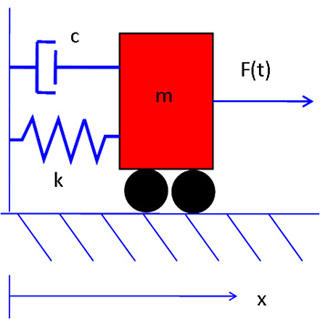2.003J | Spring 2007 | Undergraduate

# Dynamics and Control I

#### Course Description

Introduction to the dynamics and vibrations of lumped-parameter models of mechanical systems. Kinematics. Force-momentum formulation for systems of particles and rigid bodies in planar motion. Work-energy concepts. Virtual displacements and virtual work. Lagrange's equations for systems of particles and rigid bodies in …

## Course Info

##### Learning Resource Types
Problem Sets
Exams
Lecture NotesA moving cart attached to a wall by a spring and a dashpot. The equations of motion for every 1-degree-of-freedom system can be linearized around the equilibrium points to the second order differential equation that describes this system. Thus, understanding the free response and selected forced responses for this system can give deep insight into the stability of the equilibrium points and the behavior of a large number of systems. See Lectures 20 and 21 for more information. (Image by MIT OCW.)